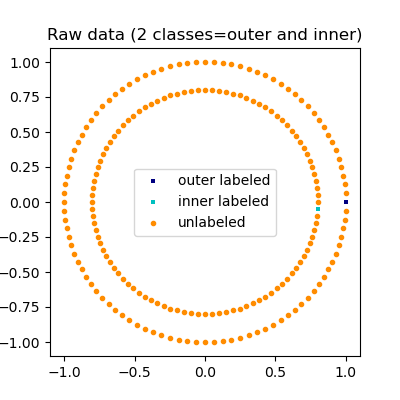# 1.14. Semi-supervised learning¶

Semi-supervised learning is a situation in which in your training data some of the samples are not labeled. The semi-supervised estimators in sklearn.semi_supervised are able to make use of this additional unlabeled data to better capture the shape of the underlying data distribution and generalize better to new samples. These algorithms can perform well when we have a very small amount of labeled points and a large amount of unlabeled points.

Note

Semi-supervised algorithms need to make assumptions about the distribution of the dataset in order to achieve performance gains. See here for more details.

## 1.14.1. Self Training¶

This self-training implementation is based on Yarowsky’s  algorithm. Using this algorithm, a given supervised classifier can function as a semi-supervised classifier, allowing it to learn from unlabeled data.

SelfTrainingClassifier can be called with any classifier that implements predict_proba, passed as the parameter base_classifier. In each iteration, the base_classifier predicts labels for the unlabeled samples and adds a subset of these labels to the labeled dataset.

The choice of this subset is determined by the selection criterion. This selection can be done using a threshold on the prediction probabilities, or by choosing the k_best samples according to the prediction probabilities.

The labels used for the final fit as well as the iteration in which each sample was labeled are available as attributes. The optional max_iter parameter specifies how many times the loop is executed at most.

The max_iter parameter may be set to None, causing the algorithm to iterate until all samples have labels or no new samples are selected in that iteration.

Note

When using the self-training classifier, the calibration of the classifier is important.

## 1.14.2. Label Propagation¶

Label propagation denotes a few variations of semi-supervised graph inference algorithms.

A few features available in this model:

• Kernel methods to project data into alternate dimensional spaces

scikit-learn provides two label propagation models: LabelPropagation and LabelSpreading. Both work by constructing a similarity graph over all items in the input dataset.An illustration of label-propagation: the structure of unlabeled observations is consistent with the class structure, and thus the class label can be propagated to the unlabeled observations of the training set.¶

LabelPropagation and LabelSpreading differ in modifications to the similarity matrix that graph and the clamping effect on the label distributions. Clamping allows the algorithm to change the weight of the true ground labeled data to some degree. The LabelPropagation algorithm performs hard clamping of input labels, which means $$\alpha=0$$. This clamping factor can be relaxed, to say $$\alpha=0.2$$, which means that we will always retain 80 percent of our original label distribution, but the algorithm gets to change its confidence of the distribution within 20 percent.

LabelPropagation uses the raw similarity matrix constructed from the data with no modifications. In contrast, LabelSpreading minimizes a loss function that has regularization properties, as such it is often more robust to noise. The algorithm iterates on a modified version of the original graph and normalizes the edge weights by computing the normalized graph Laplacian matrix. This procedure is also used in Spectral clustering.

Label propagation models have two built-in kernel methods. Choice of kernel effects both scalability and performance of the algorithms. The following are available:

• rbf ($$\exp(-\gamma |x-y|^2), \gamma > 0$$). $$\gamma$$ is specified by keyword gamma.

• knn ($$1[x' \in kNN(x)]$$). $$k$$ is specified by keyword n_neighbors.

The RBF kernel will produce a fully connected graph which is represented in memory by a dense matrix. This matrix may be very large and combined with the cost of performing a full matrix multiplication calculation for each iteration of the algorithm can lead to prohibitively long running times. On the other hand, the KNN kernel will produce a much more memory-friendly sparse matrix which can drastically reduce running times.